SBOS566C June   2017  – October 2018

PRODUCTION DATA.

1. Features
2. Applications
3. Description
1.     Device Images
4. Revision History
5. Pin Configuration and Functions
6. Specifications
7. Detailed Description
1. 7.1 Overview
2. 7.2 Functional Block Diagram
3. 7.3 Feature Description
4. 7.4 Device Functional Modes
8. Application and Implementation
1. 8.1 Application Information
2. 8.2 Typical Application
3. 8.3 Other Application Examples
9. Power Supply Recommendations
10. 10Layout
11. 11Device and Documentation Support
12. 12Mechanical, Packaging, and Orderable Information

• D|8
• DGK|8
• DGK|8

### 6.6 Typical Characteristics

at TA = 25°C, VS = ±18 V, and RL = 2 kΩ (unless otherwise noted)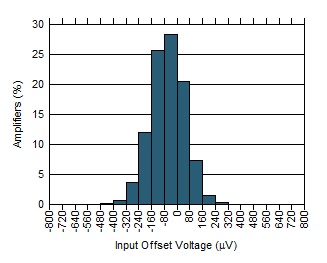N = 1160 Mean = –139.2 µV Std. Dev. = 105.4 µV
Figure 1. Input Offset Voltage Distribution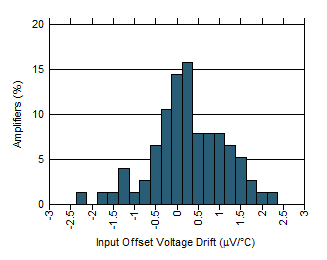Figure 3. Offset Voltage Drift Distribution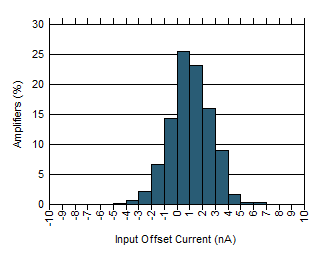N = 1160 Mean = 0.07 nA Std. Dev. = 1.58 nA
Figure 5. Input Offset Current DistributionFigure 7. Open-Loop Gain and Phase vs Frequency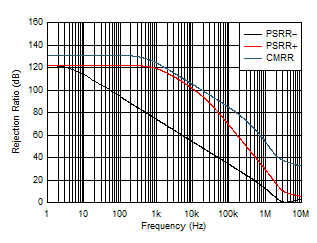Figure 9. CMRR and PSRR vs Frequency (Referred to Input)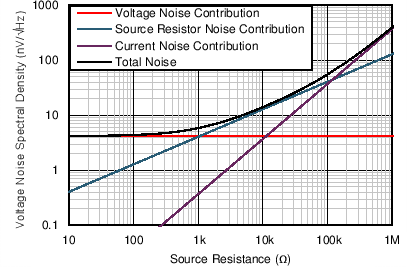Figure 11. Voltage Noise vs Source Resistance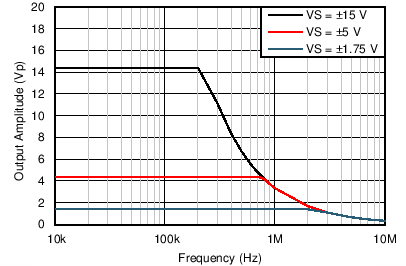Figure 13. Maximum Output Voltage vs Frequency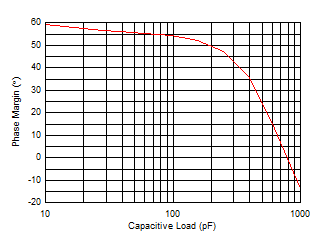G = 1
Figure 15. Phase Margin vs Capacitive Load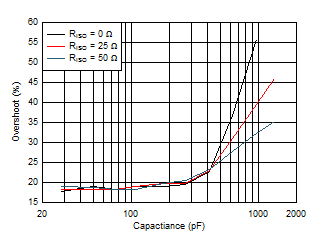G = –1 10-mV input step
Figure 17. Overshoot vs Capacitive LoadVOUT = 3 VRMS RL = 600 Ω 80-kHz bandwidth
Figure 19. THD + N Ratio vs Frequency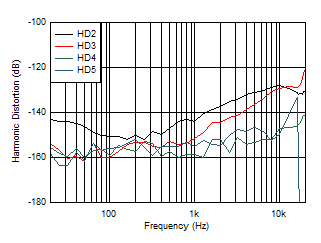VOUT = 3 VRMS G = 1 80-kHz bandwidth
Figure 21. Distortion Harmonics vs Frequency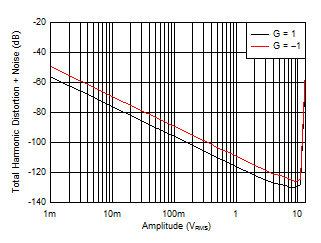f = 1 kHz 80-kHz bandwidth
Figure 23. THD + N Ratio vs Output Amplitude80-kHz bandwidth
Figure 25. Intermodulation Distortion vs Output Amplitude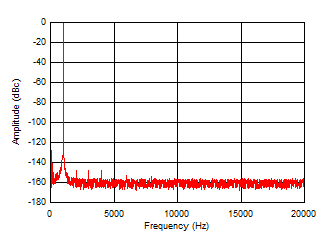f = 1 kHz VO = 3 VRMS RL = 600 Ω
Figure 27. 1-kHz Output Spectrum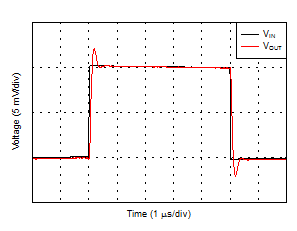100-pF capacitive load 10-mV input step G = 1
Figure 29. Small-Signal Step Response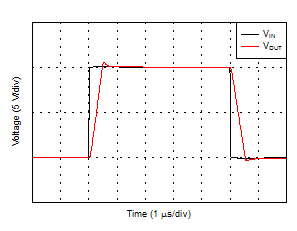100-pF capacitive load 10-V input step G = 1
Figure 31. Large-Signal Step Response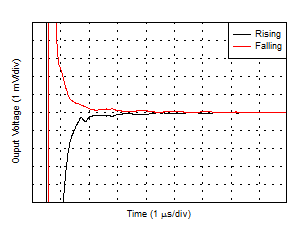Figure 33. Settling Time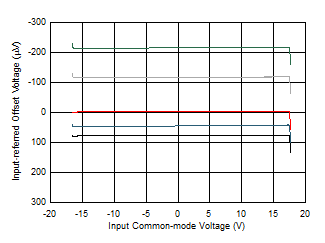5 typical units
Figure 35. Input Offset Voltage vs Common-Mode Voltage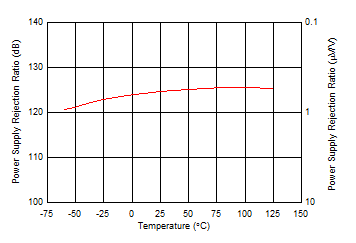Figure 37. PSRR vs Temperature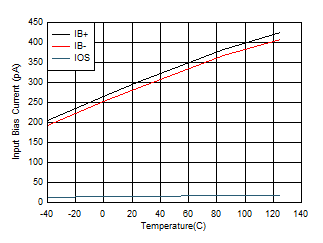Figure 39. Input Bias and Offset Current vs TemperatureVS = ±1.75 V
Figure 41. IB vs Common-Mode Voltage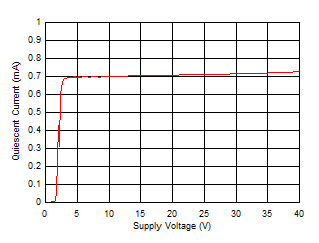Figure 43. Supply Current vs Supply Voltage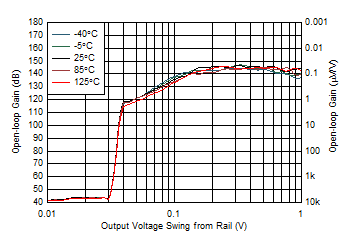VS = ±1.75 V
Figure 45. Open-Loop Gain vs Output Voltage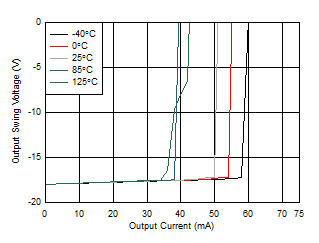Sinking
Figure 47. Output Voltage vs Output Current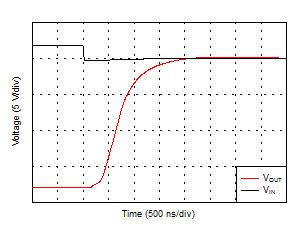Figure 49. Negative Overload Recovery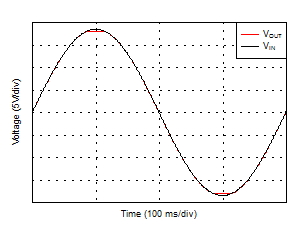Figure 51. No Phase Reversal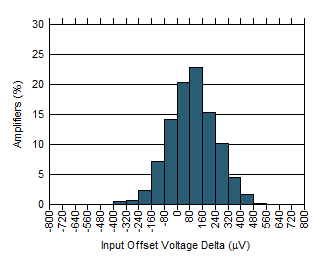N = 580 Mean = 17 µV Std. Dev. = 145.2 µV
Figure 2. Input Offset Voltage Matching (Ch. A – Ch. B)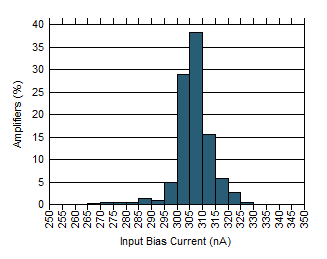N = 1160 Mean = 301.5 nA Std. Dev. = 7.03 nA
Figure 4. Input Bias Current Distribution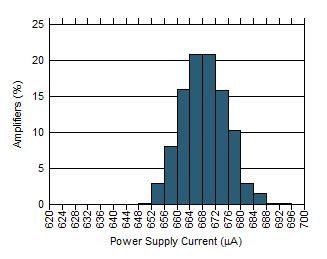N = 1160 Mean = 664.6 µA Std. Dev. = 6.98 µA
Figure 6. Power Supply Current Distribution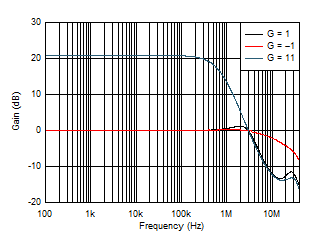Figure 8. Closed-Loop Gain vs Frequency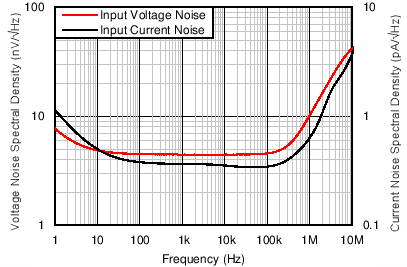Figure 10. Input Voltage Noise Spectral Density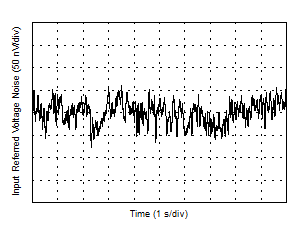Figure 12. 0.1-Hz to 10-Hz Voltage Noise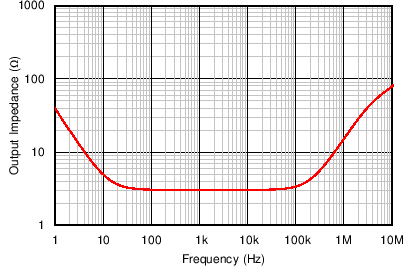Figure 14. Open-Loop Output Impedance vs Frequency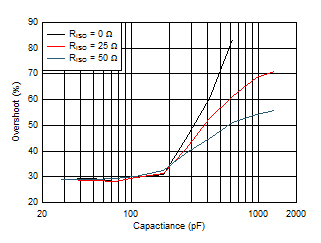G = 1 10-mV input step
Figure 16. Overshoot vs Capacitive Load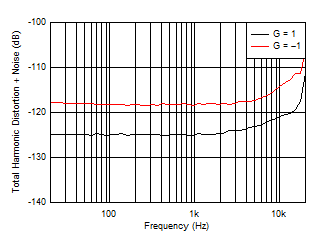VOUT = 3 VRMS 80-kHz bandwidth
Figure 18. THD + N Ratio vs Frequency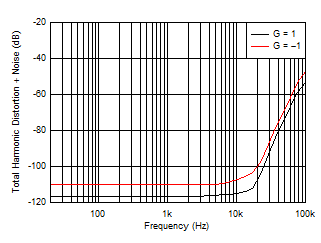VOUT = 3 VRMS 500-kHz bandwidth
Figure 20. THD + N Ratio vs FrequencyVOUT = 3 VRMS G = –1 80-kHz bandwidth
Figure 22. Distortion Harmonics vs Frequency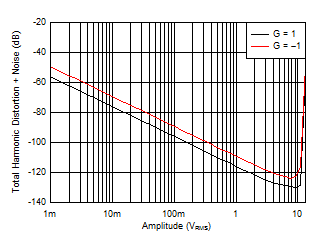f = 1 kHz RL = 600 Ω 80-kHz bandwidth
Figure 24. THD + N Ratio vs Output Amplitude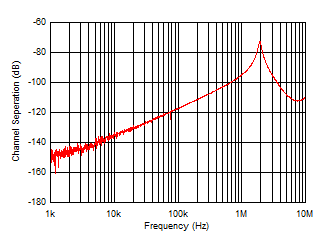Figure 26. Channel Separation vs Frequency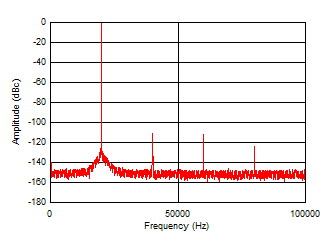f = 20 kHz VO = 3 VRMS RL = 600 Ω
Figure 28. 20-kHz Output Spectrum100-pF capacitive load 10-mV input step G = –1
Figure 30. Small-Signal Step Response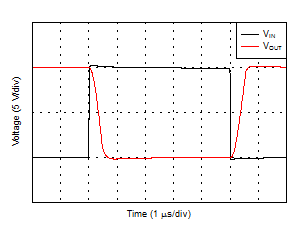100-pF capacitive load 10-V input step G = –1
Figure 32. Large-Signal Step Response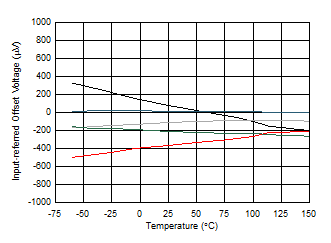5 typical units
Figure 34. Input Offset Voltage vs Temperature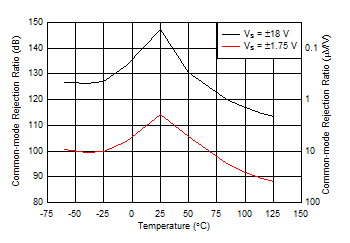Figure 36. CMRR vs Temperature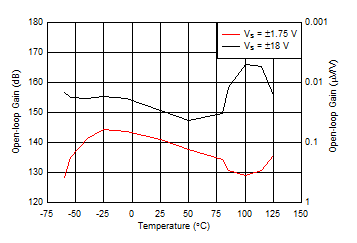Figure 38. Open-Loop Gain vs Temperature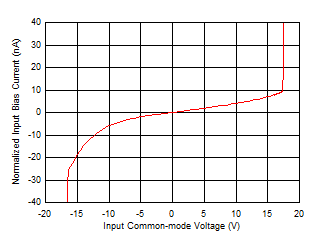Figure 40. IB vs Common-Mode Voltage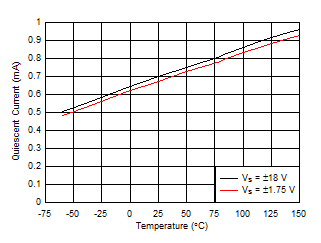Figure 42. Supply Current vs Temperature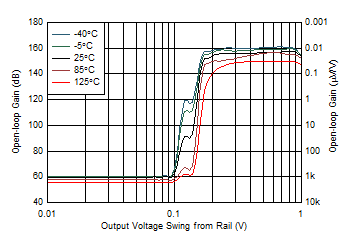Figure 44. Open-Loop Gain vs Output VoltageSourcing
Figure 46. Output Voltage vs Output Current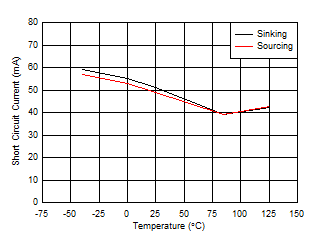Figure 48. Short-Circuit Current vs Temperature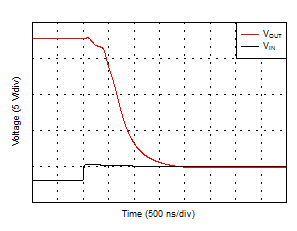Figure 50. Positive Overload Recovery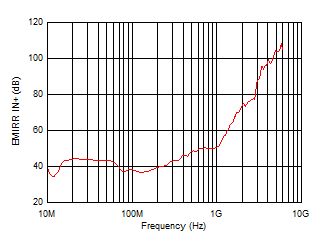Figure 52. EMI Rejection Ratio (EMIRR)